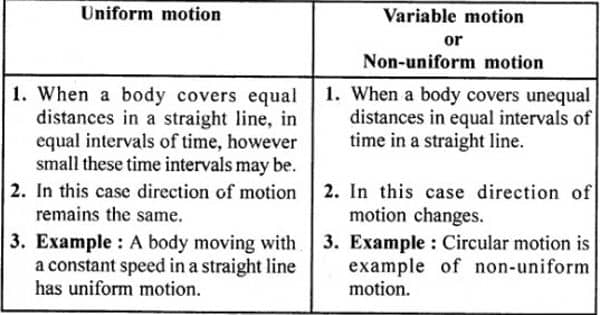Physics

# Difference between Uniform and Non-uniform MotionUniform motion is the movement of a body along a straight line with steady speed. So, the motion in which the body moves with constant speed and has zero acceleration is called Uniform motion. Non-uniform motion is the movement of an object along a straight line with variable speed. So, the motion in which the body moves with variable speed and the acceleration is not zero is called non-uniform motion.

The basic difference between uniform and non-uniform motion is that the former represents the motion in which the moving body covers the same distance at the same time, but in the case of the latter, the body travels unequal distances at the same time. For the object involving uniform rectilinear motion, the acceleration is zero, whereas the same is non-zero if the object involves non-uniform rectilinear motion.

Difference between Uniform and Non-uniform Motion

UNIFORM MOTION

• Uniform motion implies the movement of a body along with a straight line with steady speed.
• When an object moves along a straight line with a firm speed, the motion is said to be a uniform motion.
• In Physics, uniform motion is described as the motion, wherein the velocity (i.e. speed and direction) of the body traveling in a straight line remains constant.
• The object in a uniform motion covers the same distances in an equal time period.
• The speed of the object traveling in uniform motion is constant, i.e. the actual speed and the average speed of the moving body is the same.
• Example – If the speed of a car is 10 m/s, it means that the car covers 10 meters in one second. The speed is constant every second. Movement of blades of a ceiling fan.

NON-UNIFORM MOTION

• Non-uniform motion alludes to the movement of an object along a straight line with variable speed.
• Non-uniform motion, wherein the object travels along a straight line with a variable speed.
• Non-uniform motion is used to mean the movement in which the object does not cover the same distances in equal time intervals, irrespective of the length of the time intervals.
• The objects in a non-uniform motion cover dissimilar distances in an equal time period.
• The speed of the body moving in non-uniform motion varies meter by meter or kilometer by kilometer, i.e. the average speed is not equal to the average speed of the object.
• Example – If a car covers 10 meters in the first two seconds and 15 meters in the next two seconds. The motion of a train.

Information Source: